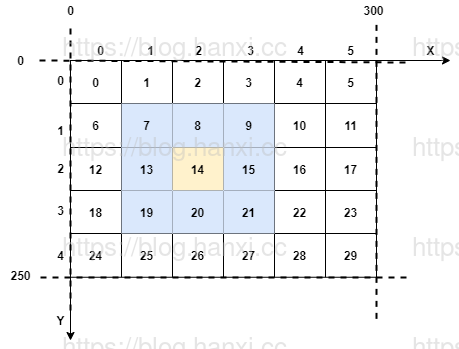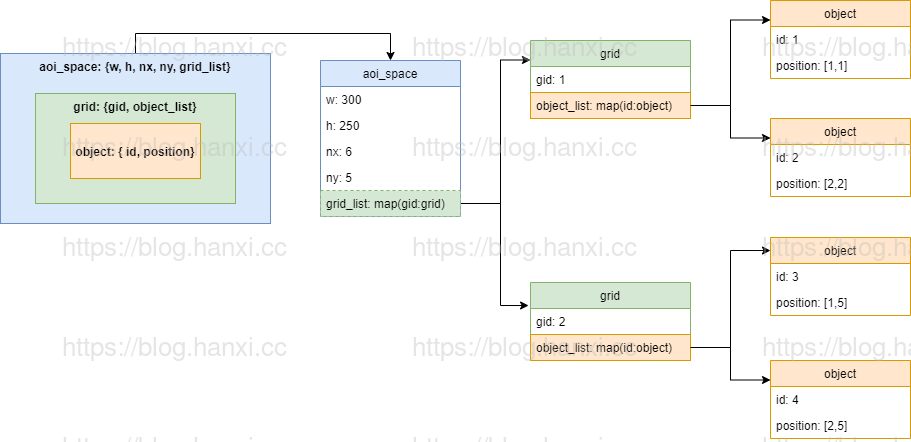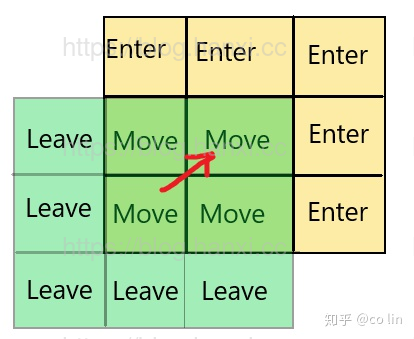AOI （Area Of Interest）算法实现

## 1. 九宫格• 场景大小： 300(w) X 250(h)
• x 轴格子数量： cntx = 6
• y 轴格子数量： cnty = 5
• 格子宽度： dw = w/cntx = 300 / 6 = 50
• 格子长度： dh = h/cnty = 250 / 5 = 50
• 格子 15 的 x 轴坐标： idx = 3
• 格子 15 的 y 轴坐标： idy = 2
• 格子编号： id = idy * cntx + idx = 2 * 6 + 3 = 15 (利用格子坐标计算给自编号)
• 格子 x 轴坐标： idx = id % cntx = 15 % 6 = 3 (利用格子 id 计算格子坐标：取余)
• 格子 y 轴坐标： idy = id % cnty = 15 // 5 = 2 (整除)
• 坐标 (255,101) 所在格子：
• idx = x/dw = 255 // 50 = 5
• idy = y/dh = 101 // 50 = 2

``````{-1,-1}, {0,-1}, {1,-1}
{-1,0},  {0,0},  {1,0},
{-1,1},  {0,1},  {1,1},
`````````struct aoi_space {
int w;
int h;
int nx;
int ny;
struct grid * grid_list;
};
struct grid {
int gid;
struct map * object_map;
};
struct object {
int id;
int position;
};```
• `grid_list` 可以直接使用数组，因为 gid 是从 0 开始且连续的。
• `object_map` 是一个 hash 表，因为 object id 是不连续的。

```// op: 'e' enter
// op: 'l' leave
// op: 'm' move
typedef void (AoiCallback)(int watcher, int marker, char op);

struct aoi_space * aoi_new(int w, int h, int nx, int ny, AoiCallback cb);
void aoi_delete(struct aoi_space * aoi);

void aoi_enter(struct aoi_space * aoi, int id, int x, int y);
void aoi_leave(struct aoi_space * aoi, int id);
void aoi_move(struct aoi_space * aoi, int id, int x, int y);```

• 没有定义视野半径，这样九宫格内的玩家都会收到消息。
• 事件消息通过返回值的方式接收。

• 进入场景：进入一个格子，取出周围9格的对象，向它们发送Enter(我)事件，同时向我发送Enter(对象)事件。
• 离开场景：取出周围9格的对象，向它们发送Leave(我)事件。
• 移动：
• 如果没跨格子，直接取9格的对象，向它们发送移动事件。
• 如果跨过格子，计算{OldGrid-NewGrid}，向它们发送Leave(我)事件，向我发送Leave(对象)事件；计算{NewGrid-OldGrid}集合，向它们发送Enter(我)事件，向我发送Enter(对象事件；计算{NewGrid*OldGrid}集合，向他们发送移动事件。• 进入场景: 根据坐标 (x,y) 计算出 id 所在的格子 ID (gid) ，找出格子里的所有对象 object_list ，然后调用 `aoi_callback(watcher, marker, 'e')` ，双方都发送 enter 事件，然后把自己加入到 grid 里。
• 离开场景： 根据坐标 (x,y) 计算出 id 所在的格子 ID (gid) ，找出格子里的所有对象 object_list ，然后调用 `aoi_callback(watcher, marker, 'l')` ，然后把自己从 grid 里删除。

Lua 代码实现在这里 https://github.com/hanxi/aoi